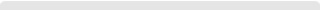#EN# 基于膜结构假定的无背索斜拉桥主梁轴力解析

## 发布时间:2021年9月6日 点击数:11200

### 1 主梁轴力解析式

#### 1.1 扇形式索面布置

hysinθ=k(L0+Lyx)h-ysinθ=k(L0+Ly-x). (1)

dNq0dx=y/tanθ+xydΝq0dx=y/tanθ+xy. (2)

dN=(1tanθ+xhksinθ(L0+Lyx))q0dxdΝ=(1tanθ+xh-ksinθ(L0+Ly-x))q0dx. (3)

NLy(x)=q0[(a+1c)(ex)+bc2ln(cx+bh)]. (4)ΝLy(x)=q0[(a+1c)(e-x)+bc2ln(cx+bh)].(4)

#### 1.2 竖琴式索面布置

k=λtλc=hsinθ(L0+Ly)k=λtλc=hsinθ⋅(L0+Ly), (5)

NLy(x)=q0(1tanθ+L0+Lyh)(L0+Lyx)ΝLy(x)=q0(1tanθ+L0+Lyh)(L0+Ly-x). (6)

#### 1.3 辐射式索面布置

NLy(x)=q0{(a+1c)ebc2[lnb+ln(ceb+1)](a+1c)x+bc2[lnb+ln(cxb+1)]}. (7)ΝLy(x)=q0{(a+1c)e-bc2[lnb+ln(ceb+1)]-(a+1c)x+bc2[lnb+ln(cxb+1)]}.(7)

{ln(ceb+1)=ceb12(ceb)2+,ln(cxb+1)=cxb12(cxb)2+. (8){ln(ceb+1)=ceb-12(ceb)2+⋅⋅⋅,ln(cxb+1)=cxb-12(cxb)2+⋅⋅⋅.(8)

NLy(x)=q0(extanθ+e2x22h)ΝLy(x)=q0(e-xtanθ+e2-x22h). (9)

### 2 主梁轴力解析式修正

NL0=η0q0L0tanα, (10)

NL1=η1q0L1tanβ, (11)

H(x)={0,x0,1,x>0. (12)Η(x)={0,x≤0,1,x>0.(12)N(x)=q0[(Lcx)1k+hkLck2ln(1kh(Lcx))]. (14)Ν(x)=q0[(Lc-x)1k+h-kLck2ln(1-kh(Lc-x))].(14)

### 3 算例分析

 主梁长度 Lc/m 近塔无索区长度 L0/m 远端无索区长度 L1/m 有索区长度 Ly/m 塔高 h/m 斜塔倾角 θ/(°) 索距比 k/m 主梁截面面积 /m2 90 10 10 70 42 52.7 0.53 9.98

 截面位置 轴力/kN 与有限元法的误差/% 有限元法 索膜法 文献方法 索膜法 文献方法 近塔处 46 130.00 47 792.00 42 604.07 3.60 7.64 L/8 45 177.89 46 343.88 40 984.54 2.58 9.28 L/4 40 039.96 40 464.05 37 088.43 1.06 7.37 3L/8 32 089.67 33 621.56 30 316.25 4.77 5.53 L/2 27 094.49 26 189.81 23 667.99 3.34 12.65

### 4 结论

1) 本文针对无背索斜拉桥结构，基于平衡荷载理论及索膜假定，考虑斜塔倾角及无索区影响，推导了多种索面布置下的主梁轴力解析式.通过算例分析，其计算结果与有限元法结果较为吻合，误差均在5%左右，对实际结果有所包络，是更偏于安全的计算方法.

2) 由本文所推导的主梁轴力解析式可知，主梁轴力与荷载、跨径、斜塔高度及索距比有关，适当增加斜塔高度，减小荷载或跨径，都可以降低主梁轴力，减小近塔处主梁截面的负担.

3) 竖琴式布索的无背索斜拉桥主梁轴力整体较大，扇形式次之，辐射式最小；但辐射式布索时轴力变化的非线性特征最明显，因此在设计时根据布索形式的不同，需要对主梁不同位置截面的材料及构造有所侧重.#### 中国华喜建筑网站

dshx@hxmjg99.com www.hxmjg.com 沪ICP备08009856号 使用条款